### Gridded data in GBIF

Gridded datasets are a known problem at GBIF. Many datasets have equally-spaced points in a regular pattern. These datasets are usually systematic national surveys or data taken from some atlas (“so-called rasterized collection designs”).

In this blog post I will describe how I found gridded dataset in GBIF.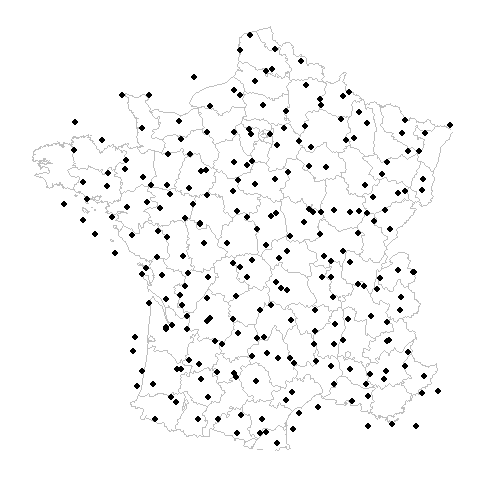### What is the problem?

Users might assume that their underlying data is reflecting a highly precise measurement of a location, but coordinates collected on a low precision might be unsuitable for species distribution modelling or some other study. Right now a user making a download at GBIF has no way of knowing if the big blob occurrence points they downloaded contains a gridded dataset. This is a problem because users might assume more precision than what is present in the download.

### How I quantified griddy-ness

After a little bit of trial and error, I arrived at a simple dataset feature (or measure):

Percentage of unique lat-long points with the most common nearest neighbor distance

# Gridded Example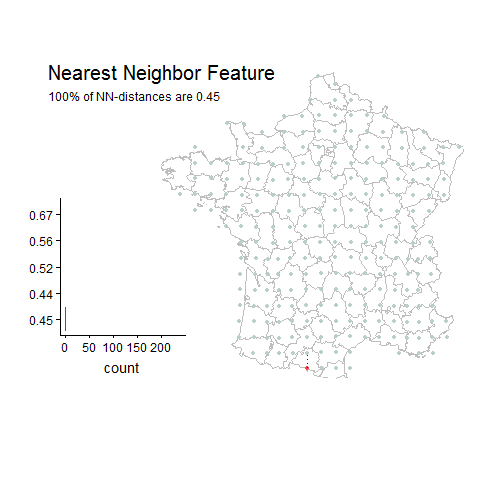# Random Example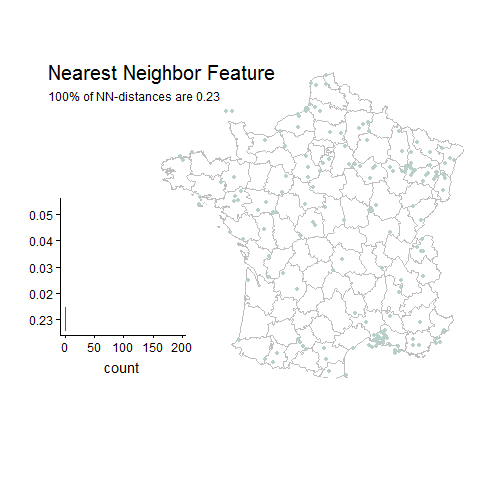• Easy to compute
• Easy to understand
• Scale independent
• Single dimensional

• Sensitive to small differences between points
• Sensitive to small datasets

### How to fix the bad things:

• Filter out small datasets
• Round distances to nearest minimum distance (I chose 0.01 decimal degrees), which is around 1 km.
• Filter out datasets with most common distances below the 0.01 minimum.
• Filter out datasets with very few remaing unique lat-long points after filtering below 0.01 minimum.

# The spectrum from gridded to random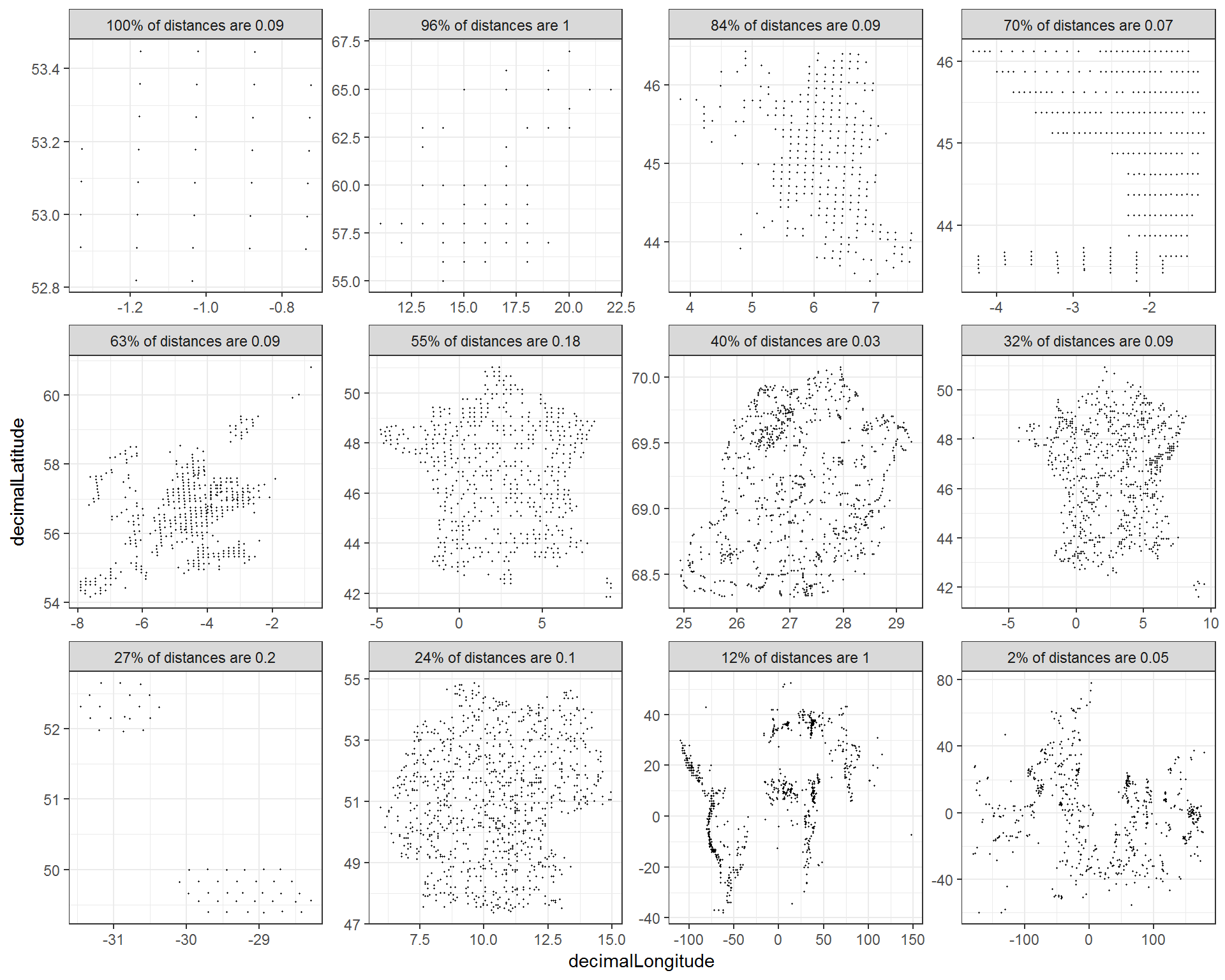### Most gridded datasets seem to be located in Europe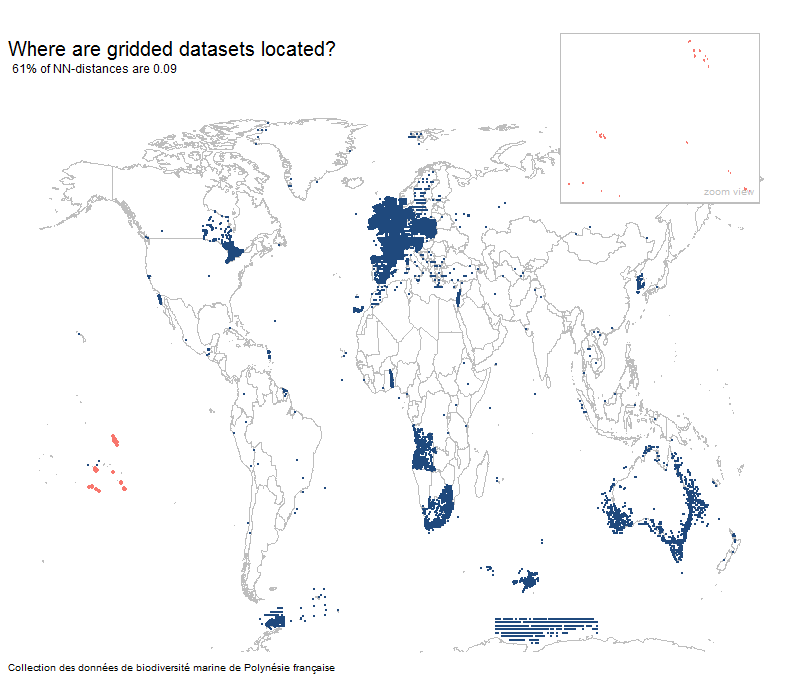## Results

• There are around 400 gridded datasets within GBIF.
• This represents around 10% of the 4000 large datasets (> 20 unique lat-long points)
• France publishes the most gridded datasets with 228 gridded datasets.
• Not all rasterized or gridded datasets are a perfect square and there is a spectrum of griddyness.
• At least 75M occurrence records are gridded above 0.01 degrees.
• Most datasets (370) are gridded at 0.1° and only 12 datasets are gridded at greater than 0.40° and 33 datasets are gridded below 0.1°.

## You can manually review all datasets that passed my filters

I have also plotted each of the datasets that have passed some basic filters here. Around 530 datasets are large enough and well sampled enough to review.

``````library(dplyr)

urlFile = 'https://raw.githubusercontent.com/jhnwllr/griddedDatasets/master/csv/griddedDataSets.csv'

griddedDataSets =
griddedDataSets %>%
filter(countNN > 30) %>% # only get datasets with a reasonable count of NN distances
filter(distanceNN > 0.02) # filter out distances close to minimum 0.01 (important!)
nrow(griddedDataSets) # datasets in the pdf``````

I have processed all occurrence datasets in GBIF and produced a griddyness score for each based off of nearest neighbor distances (described above).

### disclaimers

• Only datasets with more than 20 unique lat-long points were processed.
• Datasets were downloaded in August of 2018, so new datasets might not be in the csv.
• Mixed datasets might not be caught unless a large percentage of points are gridded.
• Only the first 1000 points were downloaded from each dataset, so large datasets that are gridded but only have a few unique lat-lon points will not be caught.
• Only looked for datasets on a grid larger than 0.01 decimal degrees.
• Not all 15k occurrence datasets are capable of being classified.
• The griddedDataSets.csv contains 4698 datasets of around 15,000 occurrence datasets with various levels of griddyness.
• Most datasets within GBIF have less than 20 unique lat-long points (from a sample of 1000 points) and are therefore difficult to classify as gridded or random.
• Even non-gridded datasets might not necessarily have an extremely precise lat-long occurrence records.

### Column definitions for griddedDataSets.csv

• datasetKey - GBIF dataset key.
• publishingCountry - Country that published the dataset.
• occCount - Total number of occurrence records in the dataset.
• datasetTitle - Title of dataset.
• percentNN - Fraction of unique lat-long points with the most common nearest neighbor distance.
• distanceNN - The most common nearest neighbor distance to the nearest 0.01 degrees.
• countNN - The count of unique lat-long points with the most common nearest neighbor distance.
• uniqueLatLon - The number of unique lat-long points in the dataset.
• numberSampled - number of points sampled from each dataset. Will be 1000 for each.

### Setting the percentNN parameter

Below is a basic example of how to filter a GBIF download in R with griddedDataSets.csv. The main variable to adjust is the percentNN or fraction of unique lat-long points with the most common distance.

• greater than 0.75 will likely remove only gridded datasets from a download, leaving all random datasets but also many gridded datasets.
• greater than 0.50 will remove most gridded datasets but few random.
• greater than 0.30 will likely remove almost all gridded datasets (recommended).
• lower than 0.30 will likely only remove mostly non-gridded datasets (too conservative).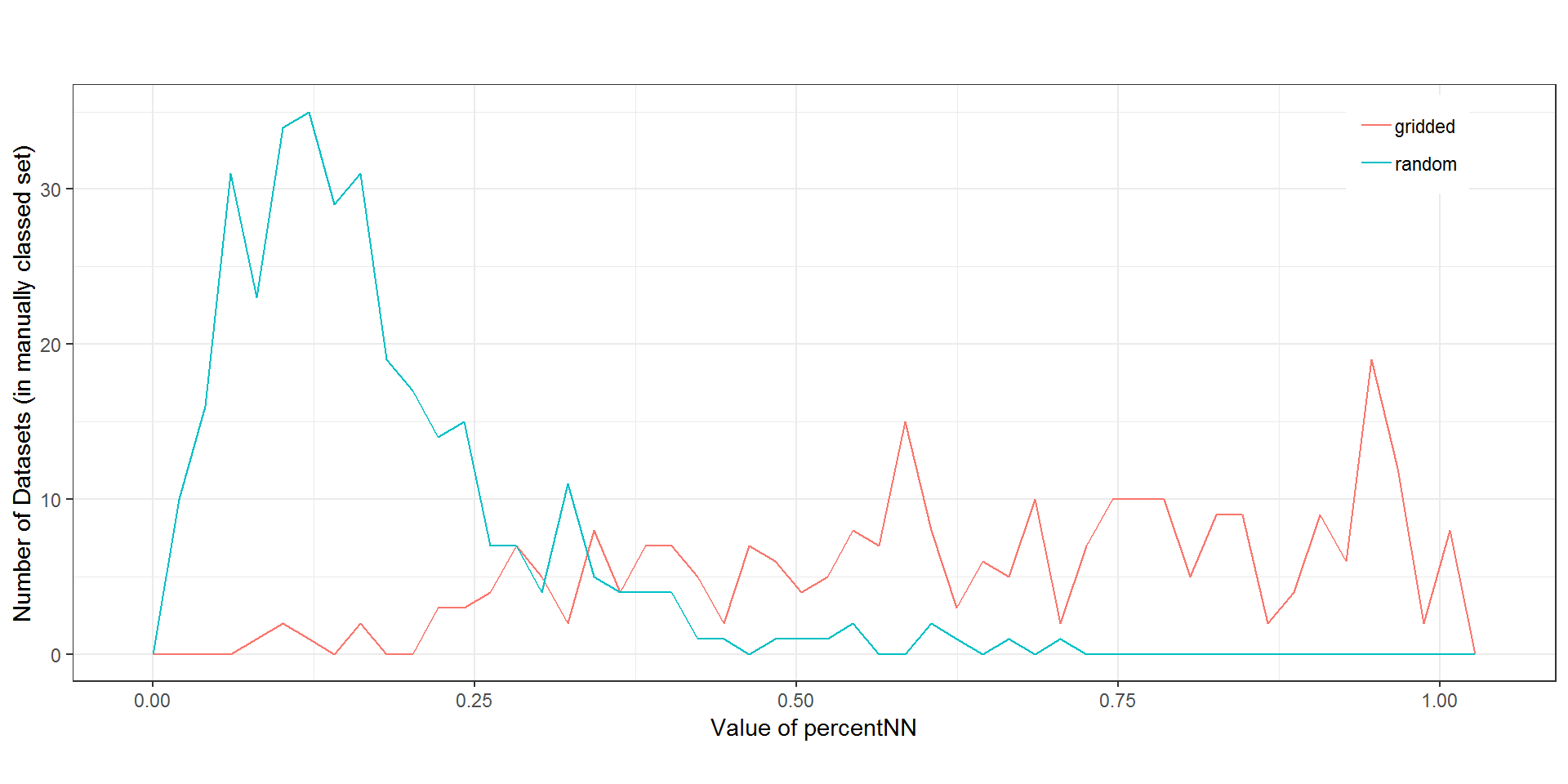## R-code to filter out gridded datasets

``````# filtering out gridded datasets

library(rgbif)
library(dplyr)

urlFile = 'https://raw.githubusercontent.com/jhnwllr/griddedDatasets/master/csv/griddedDataSets.csv'

griddedDataSets =
griddedDataSets %>%
filter(countNN > 30) %>% # only get datasets with a reasonable count of NN distances
filter(percentNN > 0.3) %>% # only filter datasets with a high percentage
filter(distanceNN > 0.02) # filter out distances close to minimum 0.01 (important!)

# Emberiza bruniceps; 500 Red-headed bunting records from France
buntingData = occ_search(taxonKey = 2491521,country = "FR",limit=500,return="data")

nrow(buntingData) # only total records 269 (September 2018)

unique(buntingData\$datasetKey) # 4 datasets

# should find 2 gridded datasets
unique(buntingData\$datasetKey) %in% griddedDataSets\$datasetKey
cleanBuntingData = buntingData[!buntingData\$datasetKey %in% griddedDataSets\$datasetKey,]

# only 4 occurrence records for Emberiza bruniceps found not in gridded datasets
nrow(buntingData) ``````

### What if a dataset is not in griddedDatasets.csv?

Below we can also check if any datasets were not in griddedDataSets.csv.

1. We can either choose to remove any dataset on in the csv (very conservative).
2. Or we can manually check each dataset in our data but not in the csv (recommended).

In this case we see that there is one dataset in our download that is not in griddedDataSets.csv.

``````# were any datasets downloaded that were not in the gridded datasets csv?

# If the dataset is not in the
unique(buntingData\$datasetKey)[!unique(buntingData\$datasetKey) %in% griddedDataSets\$datasetKey]

# 1 dataset not in griddedDataSets.csv
# 906b2d3f-dbd7-4c5c-acfc-c572c35c2b5a``````

We can plot the dataset not in griddedDataSets.csv using the GBIF map api and see it is also most likely gridded. This illustrates that not all gridded datasets will necessarily be caught by my algorithm described above, but it should be able to remove the vast majority of gridded datasets. In the case of this dataset, it was not caught because it happens to be very large and have very few unique lat-lon points.

``````library(leaflet)

prefix = 'https://api.gbif.org/v2/map/occurrence/density/{z}/{x}/{y}@1x.png?'
query = 'style=classic.poly&bin=hex&hexPerTile=30&datasetKey=906b2d3f-dbd7-4c5c-acfc-c572c35c2b5a'
tile = paste0(prefix,query)

leaflet() %>%
setView(lng = 5.4265362, lat = 43.4200248, zoom = 08) %>%

### gridded dataset not in griddedDataSets.csv

In the future, I may consider downloading a larger sample from each GBIF dataset in order to catch such cases.

# Coordiante Cleaner R package

Coordiante Cleaner is an R package written by Alexander Zizka. This package utilizes the cd_round function to find gridded or rasterized datasets. It uses a different methodology than what is described here, but is definitely worth taking a look at.

# Potential improvements

### Different Projections

Throughout this post I have implicitly been assuming all coordinates are projected using WGS84, but this might not be the case. For example, the UK uses something called Ordnance Survey National Grid, and there probably other projections that might have caused me to miss a gridded dataset.

### Multiples of the most common distance

One could imagine looking for multiples of the most common distance as a way of detecting gridded datasets. This has the potential to improve performance, but it might also not help very much since we will likely be finding datasets that are already very ‘griddy’ and square.

### Gridded datasets not sampled exactly at the same point

Near Alaska you can see that sampling seems to have occurred around the same point, but not exactly the same point.

### Looking for gridded datasets spaced smaller than 0.01 degrees

There are probably many gridded datasets at smaller than 0.01 degrees. I chose not to look for these datasets since most environmental and climate data are at a resolution equal to or higher than 0.01 degrees.

### Sampling more than 1000 records from each dataset

Probably the main issue preventing me finding all gridded datasets is low sampling. Large gridded datasets, but with very few unique lat-long points, will not be well sampled enough at 1000 records. Probably higher sampling will locate more datasets.

One might want to compute griddy-ness scores for a new download. Or we might want to increase the sampling of the datasets to be more confident that we are catching all gridded datasets. This can be done in pricipal using rgbif. In general though this process will take longer and might be difficult to acheive if there are very many datasets.

``````library(rgbif)
library(dplyr)

# key - focal key
# k - number of nearest neighbors to compute

getNNFeature = function(D,key,k) { # nearest neighbor features
D = D %>% filter(datasetKey == key)

# nearest neighbors distances
NN = FNN::get.knn(cbind(D\$decimalLongitude,D\$decimalLatitude), k=k)\$nn.dist

# Nearest Neighbor Percent Feature
minimum = 0.01
NN = round(NN,2) # round nearest neighbors nearest 0.01
# adjust precision of rounding to any desired
# NN = plyr::round_any(NN,0.01,ceiling)

TL = apply(NN,2,table) # table list
# distances less than minimum
boolL = lapply(TL,function(x) as.numeric(names(x)) > minimum)

# filter out those distances less than minimum
T = list(); for(i in 1:length(TL)) T[[i]] = TL[[i]][boolL[[i]]]

NNC = sapply(T,function(x) rev(sort(x))) # NN point count
NNPF = sapply(T,function(x) rev(sort(x))/sum(x)) # NN feature
MCD = as.numeric(names(NNPF)) # most common distances of NN

NNF = cbind(
rbind(NNC) %>% as.data.frame() %>% setNames(paste0("NNC",1:k)),
rbind(NNPF) %>% as.data.frame() %>% setNames(paste0("NNPF",1:k)),
rbind(MCD) %>% as.data.frame() %>% setNames(paste0("MCD",1:k))
)

NNF\$key = key # add key
NNF\$uniqueLatLon = nrow(D)

return(NNF) # return the nearest neighbor feature
}

# Emberiza bruniceps; 500 Red-headed bunting records from France
buntingData = occ_search(taxonKey = 2491521,country = "FR",limit=500,return="data")

keys = unique(buntingData\$datasetKey)
sampleSize = 2000 # change to increase the sample size

DL = lapply(keys,function(x) {
occ_data(datasetKey=x,hasGeospatialIssue=FALSE,hasCoordinate=TRUE,limit=sampleSize)\$data
})
occRecords = plyr::rbind.fill(DL)

# aggregate data
occRecords = occRecords %>%
group_by(datasetKey) %>%
select(decimalLatitude,decimalLongitude) %>%
count(decimalLongitude,decimalLatitude) %>% as.data.frame()

# compute the actual features
FL = list() # feature list
for(key in keys) {
# sometimes this feature fails because
# there are no points with NN dist greater than 0.01
out=try(getNNFeature(D=occRecords,key,k=4))
if(class(out) == "try-error") next
FL[[key]] = out
}
F = plyr::rbind.fill(FL) # griddyness features

griddedDataSets = F %>%
select(key,NNPF1,MCD1,NNC1,uniqueLatLon) %>%
setNames(c("datasetKey","percentNN","distanceNN","countNN","uniqueLatLon"))

# set parameters to filter with
griddedDataSets =
griddedDataSets %>%
filter(countNN > 30) %>% # only get datasets with a reasonable count of NN distances
filter(percentNN > 0.3) %>% # only filter datasets with a high percentage
filter(distanceNN > 0.02) # filter out distances close to minimum 0.01 (important!)

unique(buntingData\$datasetKey) %in% griddedDataSets\$datasetKey
cleanBuntingData = buntingData[!buntingData\$datasetKey %in% griddedDataSets\$datasetKey,] ``````